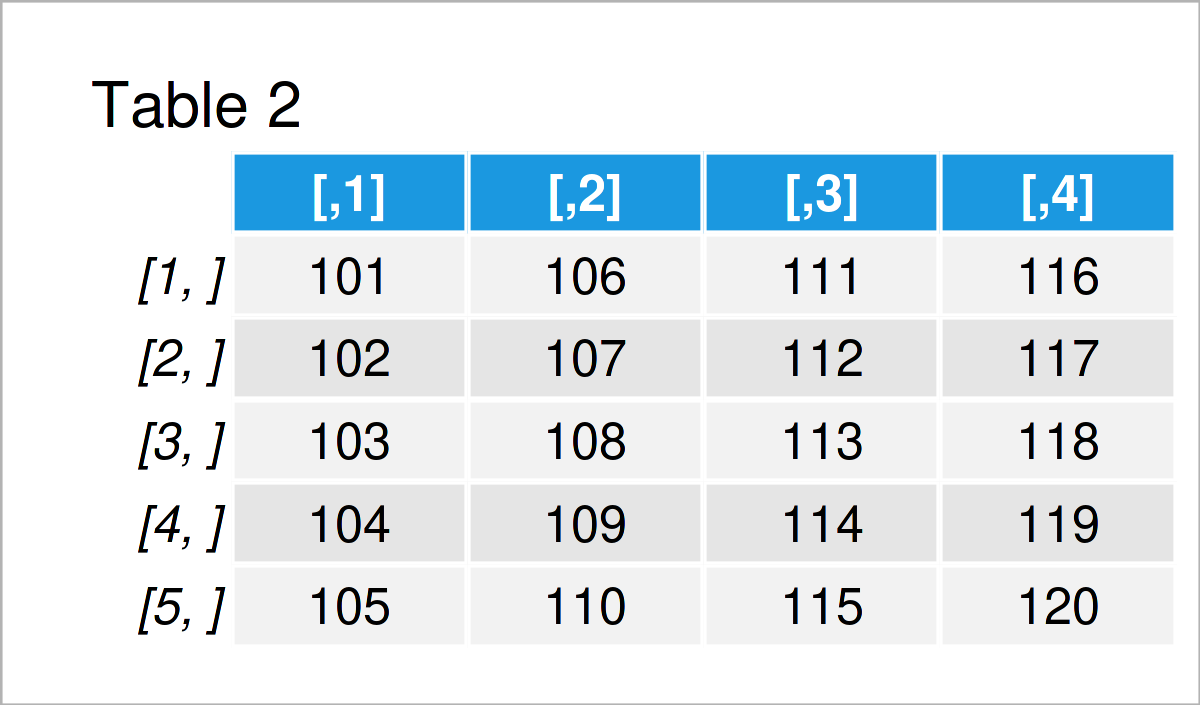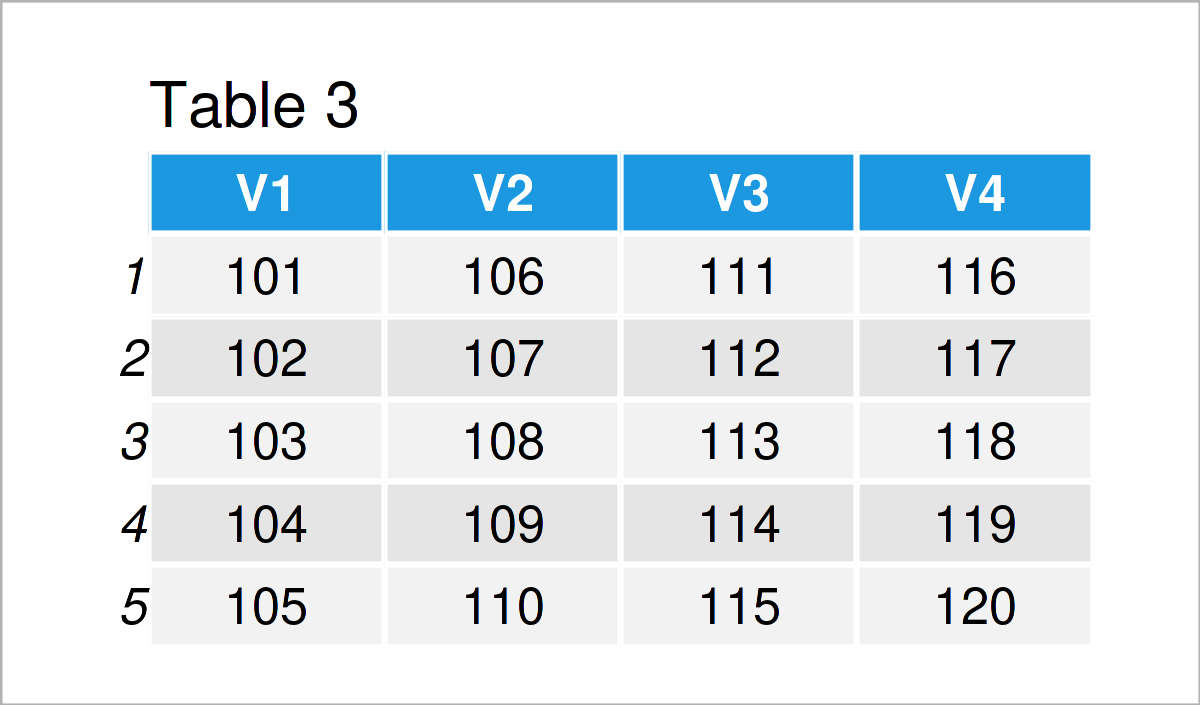# What is a Data Frame in R? (3 Examples)

In this R tutorial you’ll learn how to create and manipulate data frames.

First, the article explains what a data frame is. Then, I’ll demonstrate three examples for the handling of data frames in R. To be more specific, the tutorial consists of this:

Let’s do this!

## Data Frames Explained

A data frame is a data structure that is used to store data sets in the R programming language.

Technically speaking, data frames are lists of vectors of equal length and can contain different types of variables and data classes.

Data frames are the most common data structure in R and are provided by the basic installation of the R programming language.

The following examples demonstrate how to construct and handle data frames in R.

So without further ado, let’s move on to the R programming code!

## Example 1: Create Data Frame Using data.frame() Function

In this example, I’ll demonstrate how to create a new data frame from scratch.

For this task, we can use the data.frame function. Within the data.frame function, we have to specify the column names and the values that should be contained in each of the columns.

Have a look at the R syntax and its output below:

```data1 <- data.frame(x1 = 1:6,       # Create data frame
x2 = c("a", "v", "s", "a", "f", "f"),
x3 = "Y")
data1                               # Print data frame```The output of the previous code is shown in Table 1. We have initialized a new data frame object called data1 that contains six rows and three different columns.

## Example 2: Convert Matrix to Data Frame Using as.data.frame() Function

It is also possible to convert other data structures to the data.frame class.

In Example 2, I’ll show how to transform the matrix data type to a data frame.

For this, we first have to construct an example matrix:

```mat <- matrix(101:120, nrow = 5)    # Create matrix
mat                                 # Print matrix```Table 2 shows the output of the previous R syntax: A matrix consisting of five rows and four columns.

In the next step, we can apply the as.data.frame function to convert our matrix to the data.frame class:

```data2 <- as.data.frame(mat)         # Convert matrix to data frame
data2                               # Print data frame```As shown in Table 3, the previous syntax has created a new data frame object called data2 that contains the same values as the input matrix.

## Example 3: Import Existing Data Frame Using data() Function

The R programming language provides many pre-defined data frames that can be loaded to the current R session.

The following R programming syntax explains how to import the mtcars data set to R.

To achieve this, we can use the data() function as demonstrated below:

```data("mtcars")                      # Import data frameThe output of the previous syntax is shown in Table 4. It contains the first six rows of the mtcars data frame.

## Video, Further Resources & Summary

Do you want to learn more about the handling of data frames? Then you could have a look at the following video which I have published on my YouTube channel. I’m explaining the R programming codes of this tutorial in the video.

In addition to the video, you might have a look at the other articles on statisticsglobe.com. I have released several articles already:

At this point of the article you should have learned how to use data frame objects in the R programming language. Don’t hesitate to let me know in the comments section, in case you have any further questions. Furthermore, don’t forget to subscribe to my email newsletter in order to get updates on the newest tutorials.

Subscribe to the Statistics Globe Newsletter